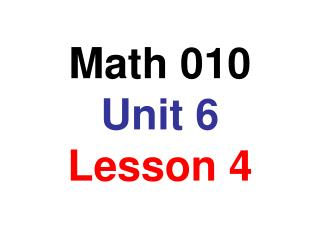DownloadDownload PresentationMath 010 Unit 6 Lesson 4

# Math 010 Unit 6 Lesson 4

Télécharger la présentation## Math 010 Unit 6 Lesson 4

- - - - - - - - - - - - - - - - - - - - - - - - - - - E N D - - - - - - - - - - - - - - - - - - - - - - - - - - -
##### Presentation Transcript

1. Math 010 Unit 6Lesson 4

2. Objectives: • to write a set in roster notation • to write a set in set-builder notation • to graph an inequality on the number line

3. Sets - the roster method The roster method of writing a set encloses a list of the elements in braces. Examples: 1. The set of the last three letters in the alphabet. {x, y, z} 2. The set of integers between 0 and 10 {1, 2, 3, 4, 5, 6, 7, 8, 9} 3. The set of integers greater than or equal to 4 {4, 5, 6, . . .}

4. Other definitions The empty set is the set that contains no elements The symbol for the empty set is { } or Æ The union of two sets, written A È B is the set that contains the elements of A and the elements of B The intersection of two sets, written A Ç B is the set that contains the elements that are common to both A and B

5. A = {3, 5, 7, 9, 11} B = { 4, 6, 8, 10, 12} C = {2, 8, 10, 14} A È B = {3, 4, 5, 6, 7, 8, 9, 10, 11, 12} B Ç C = {8, 10} A Ç C = Æ

6. Another method of representing sets is called set-builder notation. {x|x < 10, x Î positive integers} The set of all x such that x is less than 10 and x is an element of the positive integers. Read the following set: {x| x > 5, x Î integers} The set of all x such that x is greater than 5 and x is an element of the integers.

7. Write the following in set builder notation The set of negative integers greater than -100 {x | x > -100, x Î negative integers} The set of real numbers less than 3 {x | x < 3 , x Î real numbers}

8. Graph the inequality: {x | x > 1, x Î real numbers} ( -10 - 5 0 5 10 The parentheses indicate that 1 is not included in the graph. A bracket, [ , would indicate that a number is included in the graph. Another method of graphing the same inequality is shown below. -10 - 5 0 5 10

9. Graph the following: {x | x £ 2} -10 - 5 0 5 10 Graph the following: {x | x £ -1} È {x | x > 2} -10 - 5 0 5 10 Remember: The union of two graphs are the points that are in one graph or the other.

10. Graph the following: {x | x < 5} Ç {x | x > -1} -10 - 5 0 5 10 Remember: The intersection of the two graphs is the set of points they have in common

11. Solving and Graphing Inequalities

12. Linear inequalities are solved like linear equations • with one exception Simplify each side of the inequality Collect terms Divide by the coefficient of the variable • switch the inequality sign if dividing or multiplying both sides of the inequality by a negative number

13. Solve the following inequalities: x – 5 > -2 1 3 1 2 6x – £ 5x – x > -2 + 5 1 3 1 2 x > 3 6 6x – £ 5x – 36x – 2 £ 30x – 3 5x – 9 < 4x + 3 36x – 30x £ -3 + 2 5x – 4x < 3 + 9 6x £ -1 1 6 x < 12 - x £

14. Solve the following inequalities: -3x > -9 4 5 7 6 - x < x < 3 30 4 5 7 6 - x < -24x < 35 3x – 9 < 8x + 11 35 24 - x > 3x – 8x < 11 + 9 -5x < 20 x > -4

15. Words and Symbols at least at most ³ £ A basketball team must win at least 60% of their remaining games to qualify for the playoffs. They have 17 games left. How many must they win? x ³ .6(17) Let x = games they must win x ³ 10.2 The team must win at least 11 games

16. Solve the inequality and graph the solution -8x > 8 x < -1 ) -10 - 5 0 5 10 or -10 - 5 0 5 10